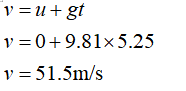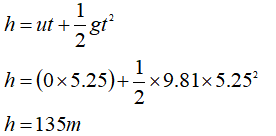# A rock is dropped from a bridge to the water below. It takes 5.25 deconds for the rock to hit the water. What is the velocity of the rock as it hits the water in metres per second? How far from the water is the bridge (in units of metres to 3 decimal places)?State at the start of the problem which direction is positive (using the up arrow or down arrow)Make a list of all given values, units, and variables they represent. Write the general form of the equation you are going to use. Insert the known variables and solve.

Question
4 views

A rock is dropped from a bridge to the water below. It takes 5.25 deconds for the rock to hit the water. What is the velocity of the rock as it hits the water in metres per second? How far from the water is the bridge (in units of metres to 3 decimal places)?

State at the start of the problem which direction is positive (using the up arrow or down arrow)

Make a list of all given values, units, and variables they represent. Write the general form of the equation you are going to use. Insert the known variables and solve.

check_circle

star
star
star
star
star
1 Rating
Given

Time of flight t = 5.25 s

Initial velocity u = 0 m/s

Solution

let us consider the downward direction as positive direction

Final velocityHeight of the bridge...

### Want to see the full answer?

See Solution

#### Want to see this answer and more?

Solutions are written by subject experts who are available 24/7. Questions are typically answered within 1 hour.*

See Solution
*Response times may vary by subject and question.
Tagged in

### Physics